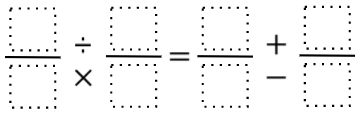# Equivalent Expressions with Fractions

Directions: Using the digits 0 to 9 at most one time each and choosing either multiplication/division or addition/subtraction, place a digit in each box to make a true statement.### Hint

Have you considered fractions greater than one?

6/9 ÷ 4/3 = 1/2 + 0/7
6/9 x 3/4 = 1/2 + 0/7
3/4 ÷ 6/9 = 1/2 + 5/8
3/4 x 9/6 = 1/2 + 5/8
3/4 ÷ 6/9 = 2/1 – 7/8
3/4 x 9/6 = 2/1 – 7/8
6/9 x 0/7 = 4/8 – 1/2

Source: Brian Errey

## Solving One-Step Inequalities with Addition

Directions: Using the digits 0 to 9 at most one time each, place a digit …

### One comment

1.